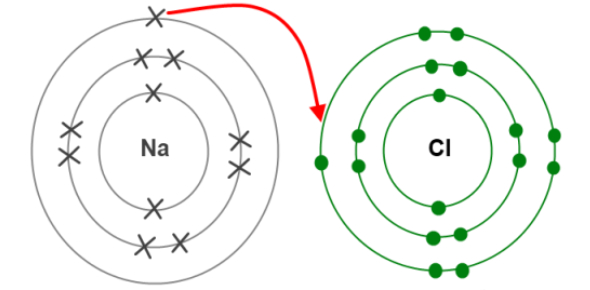# Ionic Bonding Exam: Quiz!

19 Questions | Attempts: 472
ShareSettings.

• 1.
The atomic number of magnesium is 12. When magnesium reacts to form an ionic compound, the electronic configuration of the magnesium ion formed is
• A.

2, 8

• B.

2, 8, 1

• C.

2, 8, 2

• D.

2, 8, 3

• 2.
A crystal of sodium chloride is held together by:
• A.

Covalent bonds

• B.

Double bonds

• C.

Positive ions in a "sea of electrons"

• D.

The attraction of oppositely charged ions

• 3.
How does a magnesium atom form a bond with an oxygen atom?
• A.

By giving one pair of electrons to the oxygen atom.

• B.

By sharing one pair of electrons, both electrons provided by the magnesium atom.

• C.

By sharing two pairs of electrons, both pairs provided by the oxygen atom.

• D.

By sharing two pairs of electrons, each atom donating one pair of electrons.

• 4.
Which pair of elements will combine to form an ionic compound?
• A.

Carbon and chlorine

• B.

Fluorine and sodium

• C.

Hydrogen and oxygen

• D.

Oxygen and carbon

• 5.
Element X has an electronic structure 2, 8, 8, 1. Element Y has an electronic structure of 2, 8, 6. What is the chemical formula of the compound formed between X and Y?
• A.

X2Y

• B.

XY2

• C.

XY

• D.

XY3

• 6.
What is the formula for Magnesium Oxide?
• 7.
What is the formula for Aluminum Nitride?
• 8.
What is the formula for Lithium Iodide?
• 9.
What is the formula for Sodium Fluoride?
• 10.
What is the formula for Beryllium Oxide?
• 11.
What is the name of the compound AlCl3?
• 12.
What is the name of the compound Mg3N2?
• 13.
What is the name of the compound CaO?
• 14.
What is the name of the compound HI?
• 15.
What is the name of the compound NiO?
• 16.
What is the name of the compound NaBr?
• 17.
What is the name of the compound KCl?
• 18.
What is the formula for Mercury Oxide?
• 19.
What is the formula for lithium hydroxide?

## Related TopicsBack to top
×

Wait!
Here's an interesting quiz for you.